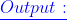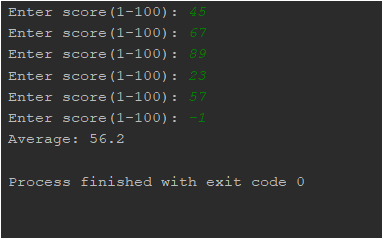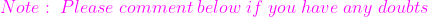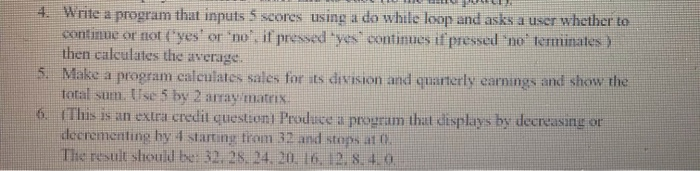Question

# Write a program that uses a while loop to read in a series of test scores,...

Write a program that uses a while loop to read in a series of test scores, then calculates the average. Control the number of repetitions by using a sentinel value.

In python ty

```score = int(input("Enter score(1-100): "))
total = 0
count = 0
while not(score<1 or score>100):
total += score
count += 1
score = int(input("Enter score(1-100): "))
if(count==0):
print("No valid scores entered")
else:
print("Average:",(total/count))```#### Earn Coins

Coins can be redeemed for fabulous gifts.

Similar Homework Help Questions
• ### Write a program that allows the user to enter a series of exam scores. The number...

Write a program that allows the user to enter a series of exam scores. The number of scores the user can enter is not fixed; they can enter any number of scores they want. The exam scores can be either integers or floats. Then, once the user has entered all the scores they want, your program will calculate and print the average of those scores. After printing the average, the program should terminate. You need to use a while loop...

• ### Write a C++ program that uses a do or a while loop that has the user...

Write a C++ program that uses a do or a while loop that has the user enter golf scores, terminated by a zero. After the zero has been entered, display the number of scores entered and their average.

• ### (While-loop controlled by a sentinel value) Write a program that reads an unspecified number of integers....

(While-loop controlled by a sentinel value) Write a program that reads an unspecified number of integers. Your program ends with the input 0. Determine and display how many positive and negative values have been read, the maximum and minimum values, and the total and average of the input values (not counting the final 0).

• ### 1- Write a program to read 10 numbers and find the average of these numbers. Use...

1- Write a program to read 10 numbers and find the average of these numbers. Use a while loop to read these numbers and keep track of input numbers read. Terminate the while loop with a sentinel value. 2- Now modify the program to find the maximum of these numbers as well. The program should print the number of elements read as input and run until -1 is entered. (-1 is the sentinel that terminates the loop and the program)...

• ### C++ 4. Write a program that inputs 5 scores using a do while loop and asks...C++ 4. Write a program that inputs 5 scores using a do while loop and asks a user whether to continue or not ("yes" or "no il pressed you continues if pressed no' terutiinales) then calculates the average. 5. Make a program calculates sales for its division and quarterly earnings and show the total sum. Use 5 by 2 array matrix 6. This is an extra credil questioni Produce a program that displays by decreasing or decremeniting by 4 starting...

• ### In C, write a program to read 5 student scores from keyboard the average of test...

In C, write a program to read 5 student scores from keyboard the average of test score. Use for loop. Instead of entering the 5 scores each time you run the program, you can use i/o redirect. Create an input file inputfor.txt, then add 5 scores in it. Run the command like this: scorefor < inputfor.txt

• ### Objectives: Use the while loop in a program Use the do-while loop in a second program...Objectives: Use the while loop in a program Use the do-while loop in a second program Use the for loop in a third program Instructions: Part A. Code a for loop to print the Celsius temperatures for Fahrenheit temperatures 25 to 125. C = 5/9(F – 32).Output should be in a table format: Fahrenheit Celsius 25 ? . . . . . . . . Turn in the source and the output from Part A. Part B. Code a while...

• ### Write a C++ program. Using the while loop or the do – while loop write a...

Write a C++ program. Using the while loop or the do – while loop write a program that does the following: Calculate the average of a series of homework grades (0 - 100) entered one at a time. In this case the lowest score will be dropped and the average computed with the remaining grades. For example suppose you enter the following grades: 78, 85, 81, 90, 88, 93 and 97.The average will be computed from the 6 grades 85,...

• ### Write a Python named numbersread.py that uses a loop to read and process numbers.txt. The program...

Write a Python named numbersread.py that uses a loop to read and process numbers.txt. The program should output all of the integers, one per line, and then output the total of the even integers and the total of the odd integers.